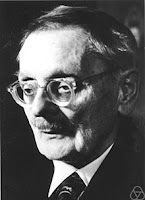## Thursday, July 4, 2019

### Mathematical theologyErnst Zermelo
Ernst Zermelo (1871-1953) was a famous mathematician of the early twentieth century. Among his achievements, the following can be mentioned:
• In 1899 he discovered Russell’s paradox, two years before Russell. Although he did not publish it, he did comment it with his colleagues at the University of Göttingen, such as David Hilbert. Russell’s paradox proved that Cantor’s set theory is inconsistent, since it makes it possible to build the set of all sets that don’t belong to themselves. There are sets that don’t belong to themselves, such as the set of even numbers, which is not an even number. Others do belong to themselves, such as the set of infinite sets, which is an infinite set. Now we can ask ourselves: Does the set of all the sets that don’t belong to themselves belong to itself? This question leads us to a paradox: if it does belong, it must belong; and if it doesn’t belong, it mustn’t belong.
• In 1904 he proved the well-ordering theorem as the first step towards proving the continuum hypothesis, the first of Hilbert’s 23 unsolved problems. The well-ordering theorem states that every set can be well-ordered, which means that any non-empty ordered subset must have a minimum element. To prove it, he proposed the axiom of choice, which we will discuss later.
• In 1905 he began to work on an axiomatic set theory. His system, improved in 1922 by Adolf Fraenkel, is a set of 8 axioms, which today is called the Zermelo-Fraenkel (ZF) system. Adding the axiom of choice to this system, we obtain the ZFC system, which is most used today in set theory.
What does the axiom of choice say? This:
The Cartesian product of a collection of non-empty sets is non-empty.
In other words: if we have a certain number of sets, all of which have at least one element, it is possible to build a set by taking an element from each of the previous sets.
It seems a truism, and if the number of those sets is finite, it is clear that it is true. But what happens if the number of sets is infinite? Then it’s not so obvious. For some time, many mathematicians refused to accept the axiom of choice, although today almost everyone accepts it.Bertrand Russell
Bertrand Russell proposed an illustration of the axiom of choice that explains why it is so called. Suppose we have an infinite set of boxes each of which contains a pair of shoes. We can build a new set, with one shoe of each pair, by choosing from each set (for instance) the left foot shoe. On the other hand, if we have an infinite set of boxes with pairs of socks, where we don’t know which sock corresponds to the left foot and which to the right foot, we don’t see at first sight how that selection can be made. Zermello's axiom of choice asserts that we can always find a selection criterion (although it does not tell us what it is) that makes it possible to build a new set with one sock of every pair.
From the axiom of choice follows Zorn’s Lemma, which states that every partially ordered set, in which every totally ordered non-empty subset has an upper bound, contains at least one maximal element.
Explanation: In a partially ordered set, you can define a sorting criterion (≤) that decides how elements should be ordered, although that criterion does not have to apply to all possible pairs of elements in the set. If it can be applied to every pair of elements, the set is totally ordered. An upper bound of a set is an element that is greater than or equal to all the others in the set, according to the sorting criterion. The maximal element is one that is not less than any other.
The mathematical journal Manifold of the University of Warwick, number 6 (1970, pp. 50-51) contains an article by Vox Fisher entitled Ontology Revisited, which proves the existence of God using the axiom of choice. This is the proof:
Theorem: The axiom of choice is equivalent to the existence of a unique God.
Proof: Consider the set of all the properties of all beings. The subsets of that set can be ordered by inclusion. This ordering is partial, for given a pair of subsets, one subset is not always included in the other. According to Zorn’s Lemma, the set in question must contain at least one maximal element. Let’s call this element God. On the other hand, Existence is one of the properties of some beings. The following is obviously true:
God Í God È {Existence}
But if God is a maximal element, this element can’t be less than any other element, thus:
God = God È {Existence}
Therefore God has the property of existence. Ergo God exists.
The remainder of the article proves the uniqueness of the maximal element, i.e. that God is unique.
It can be seen that this mathematical proof of the existence of God is equivalent to St. Anselm’s ontological proof (hence the title of the article). It is curious that, although St. Anselm’s proof is not currently accepted by many philosophers, it is well seen by some mathematicians. Kurt Gödel’s mathematical proof for the existence of God is also based on the ontological proof, although it follows a different procedure.

The same post in Spanish
Thematic Thread on Science and Religion: Previous Next
Thematic Thread on Mathematics: Previous Next
Manuel Alfonseca